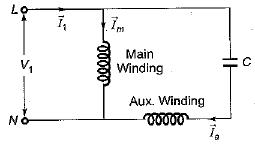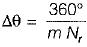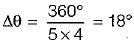Courses

# Fractional KW Motors of Electrical Machines - 2

## 20 Questions MCQ Test Topicwise Question Bank for Electrical Engineering | Fractional KW Motors of Electrical Machines - 2

Description
This mock test of Fractional KW Motors of Electrical Machines - 2 for Electrical Engineering (EE) helps you for every Electrical Engineering (EE) entrance exam. This contains 20 Multiple Choice Questions for Electrical Engineering (EE) Fractional KW Motors of Electrical Machines - 2 (mcq) to study with solutions a complete question bank. The solved questions answers in this Fractional KW Motors of Electrical Machines - 2 quiz give you a good mix of easy questions and tough questions. Electrical Engineering (EE) students definitely take this Fractional KW Motors of Electrical Machines - 2 exercise for a better result in the exam. You can find other Fractional KW Motors of Electrical Machines - 2 extra questions, long questions & short questions for Electrical Engineering (EE) on EduRev as well by searching above.
QUESTION: 1

### A single phase induction motor is

Solution:

A single-phase IM is not self starting, therefore an auxilary winding is used to start this motor.

QUESTION: 2

### The capacitor in a capacitor-start induction run ac motor is connected in series with

Solution:Here, C is connected in series with auxiliary winding (or starting winding).

QUESTION: 3

### Which of the following statements associated with shaded pole induction motor is incorrect?​

Solution:
QUESTION: 4

Assertion (A) : In a single phase induction motor, while the motor is connected to single phase ac supply and is rotating, the two fields (stator and rotor field) combine to form a resultant rotating field revolving at a synchronous speed.
Reason (R) : The rotor field created by rotor currents acts at right angle to the stator field and also lags the stator field by 90° electrical degrees in time phase.

Solution:
QUESTION: 5

Assertion (A) : Linear induction motor wide use in industry and electric traction.
Reason (R) : Linear induction motors are cheaper in initial as well as in maintenance costs.

Solution:

Assertion is true because linear motion induction motors have linear motion due to which they are wide used in industry and electric traction.

QUESTION: 6

In comparison to a resistance start motor, the capacitor start motor has
1. high starting torque
2. high running torque
3. larger phase difference between two winding currents
4. low starting current
​Out of these, the correct answer is

Solution:
QUESTION: 7

The direction of rotation of a shaded pole induction motor

Solution:
QUESTION: 8

In various types of split-phase, 1-phase induction motors, the starting torques produced are in the following descending order.

Solution:

Shaded pole motor has smallest starting torque and spe e d (1 - 2 rpm) .Tst of capacitor-split phase IM > Tst of resistance-split phase IM.

QUESTION: 9

Which of the following statements associated with an ac series motor is incorrect?

Solution:
QUESTION: 10

Speed of universal motor is

Solution:
QUESTION: 11

A single phase reluctance motor

Solution:
QUESTION: 12

The torque of a reluctance motor can be effectively decreased by

Solution:

Torque developed in a reluctance motor is given by
Te ∝ (Rlq - Rld)
If  Rlq/Rld is less, it means Rlq < RId and thus, Te is less.

QUESTION: 13

In a single phase hysteresis motor

Solution:

In a 1-ϕ hysteresis motor, eddy current torque, Teddy ∝ s and hysteresis
torque = constant
During starting, slip is high, therefore torque developed is due to both eddy current and hysteresis. However, during running condition slip is very low due to which eddy current torque will be very less. Therefore, torque is only due to hysteresis during running condition.

QUESTION: 14

Match List-I (Type of motors) with List-ll (Application) and select the correct answer using the codes given below the lists:
List-I
A. Shaded pole motor
B. Reluctance motor
C. Universal motor
D. Single phase IM
List-ll
1. Ceiling fan
2. Food mixture
3. Timing and control purposes
4. Toys
Codes:
A B C D
(a) 4 3 2 1
(b) 2 3 1 4
(c) 3 4 2 1
(d) 2 1 3 4

Solution:
QUESTION: 15

Which of the following single phase motor has no teeth or winding?

Solution:
QUESTION: 16

Match List-I (Type of motors) with List-ll (Characteristics) and select the correct answer using the codes given below the lists:
List-I
A. Shaded Pole motor
B. Hysteresis motor
C. PMDC motor
D. Capacitor start motor
List-ll
1. Least noise
2. Highest starting torque
3. Lowest speed
4. Used in cassette tape recorder
Codes:
A B C D
(a) 3 4 2 1
(b) 1 4 2 3
(c) 3 1 4 2
(d) 1 2 3 4

Solution:

Capacitor start motor has highest starting toque. Hysteresis motor gives noise less operation.

QUESTION: 17

Assertion (A): When a ceiling fan using a capacitor run motor is switched on, it hums but does not run. When it is driven by some external means it runs in the direction in which it was made to run.
Reason (R): The capacitor connected in series with the auxiliary winding is short circuited.

Solution:

The capacitor might be open circuited due to which no starting torque is developed and motor fails to start.

QUESTION: 18

The rotor of a stepper motor has

Solution:
QUESTION: 19

A stepping motor has four concentric coils on its 4-pole stator. It has an unwound variable reluctance motor with 5 teeth. The step angle for this motor is

Solution:

Step size/angle,Given,
Nr = Number of rotor teeths = 5
m = Number of phase/stacks = 4
∴QUESTION: 20

A single phase induction motor is running at N rpm. Its synchronous speed is Ns. If its slip w.r.t forward field is s. What is the slip w.r.t. the backward field?

Solution:

Sf= s
Sb=(Ns+N)/Ns
= 1+1-s
=2-s ( As N/Ns = 1-s )